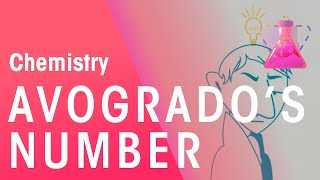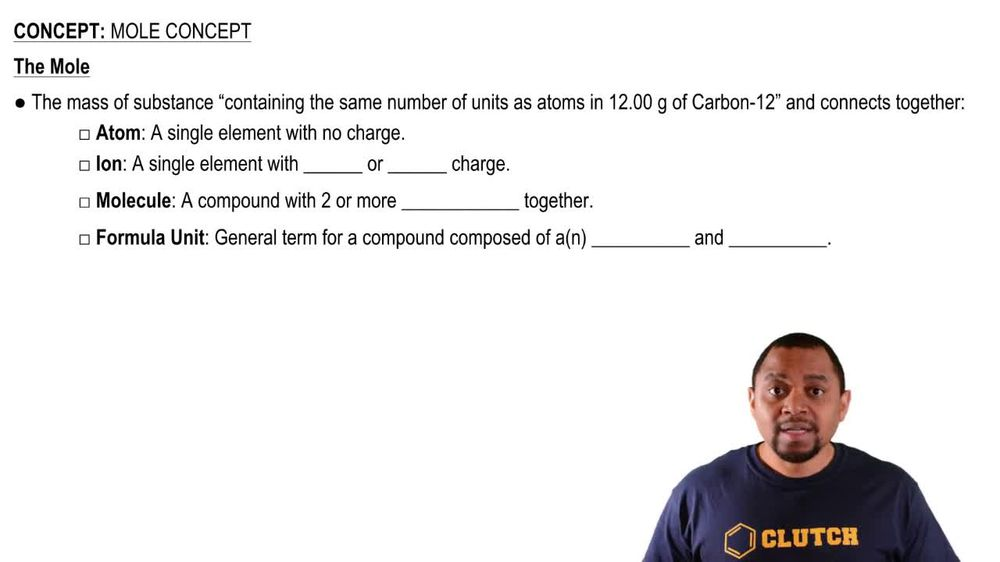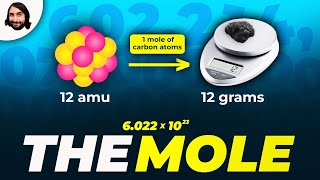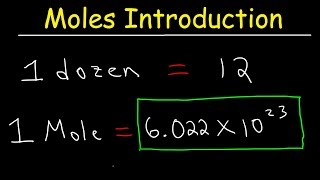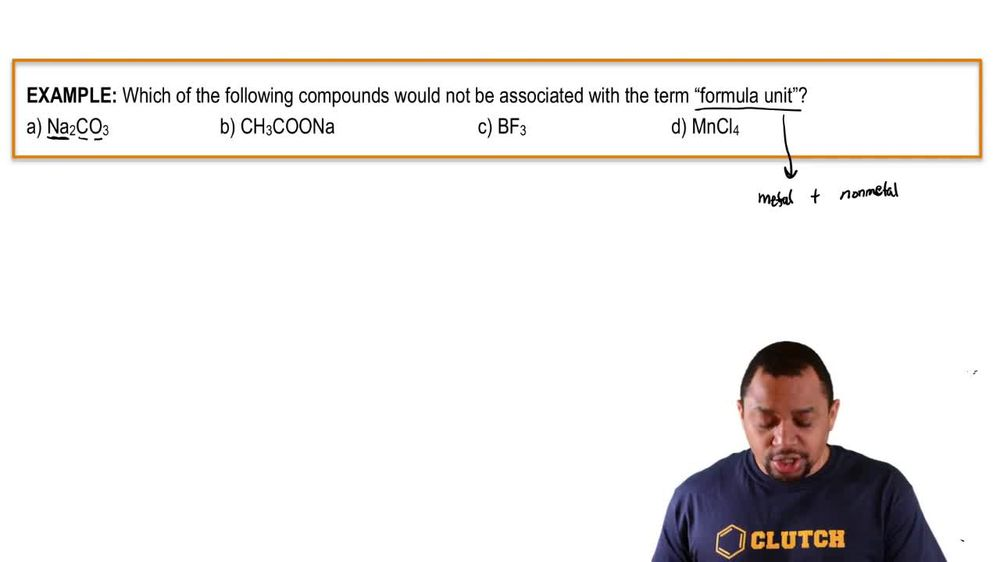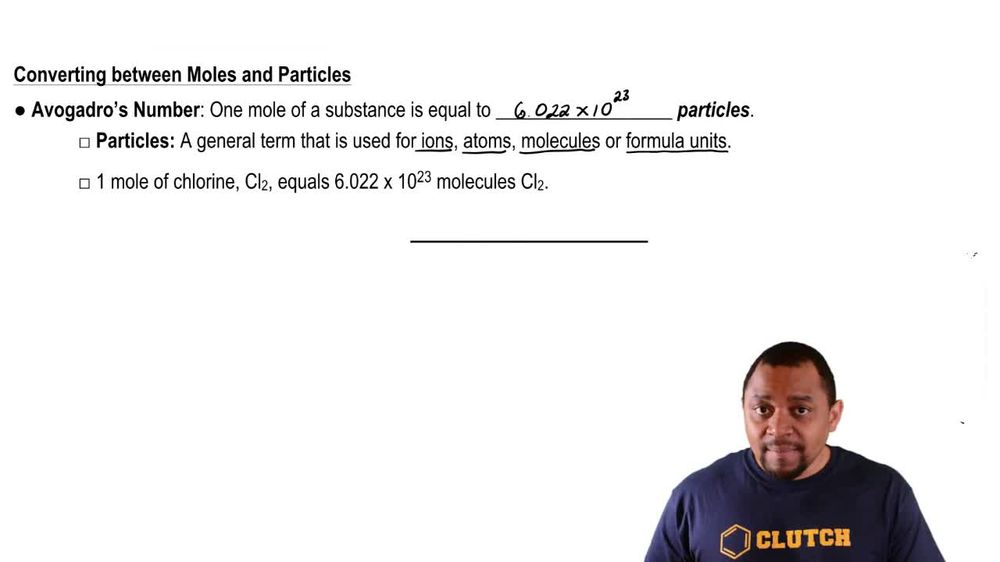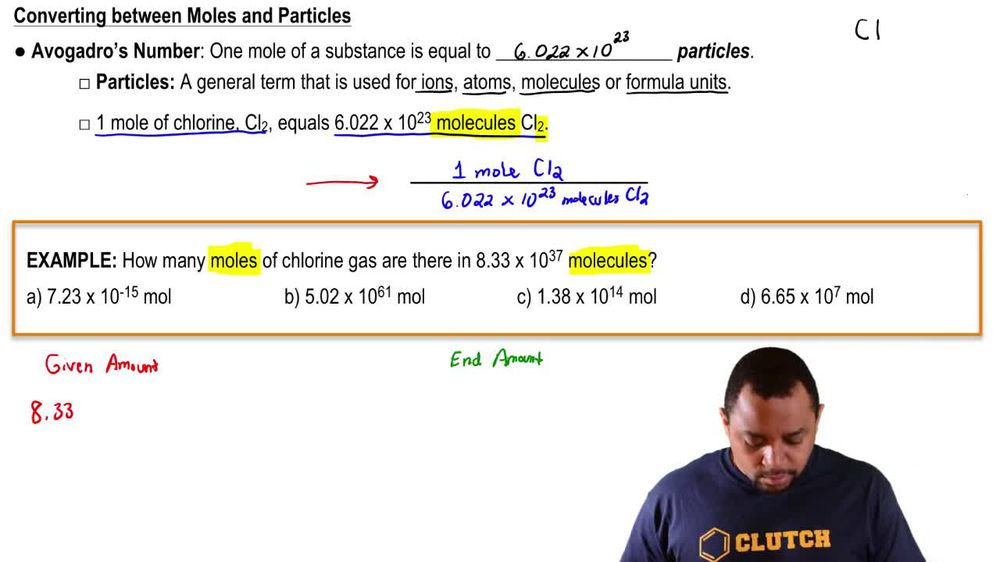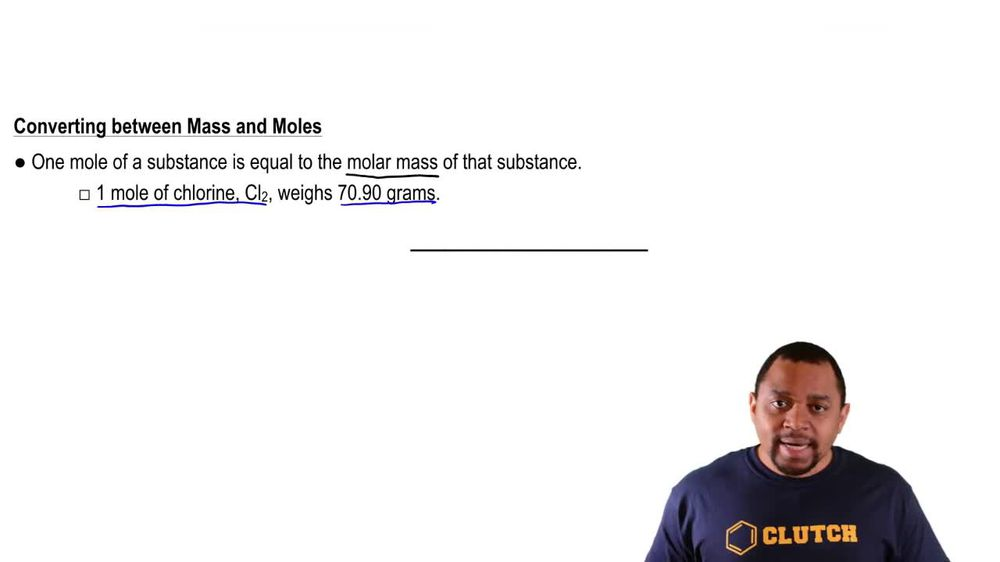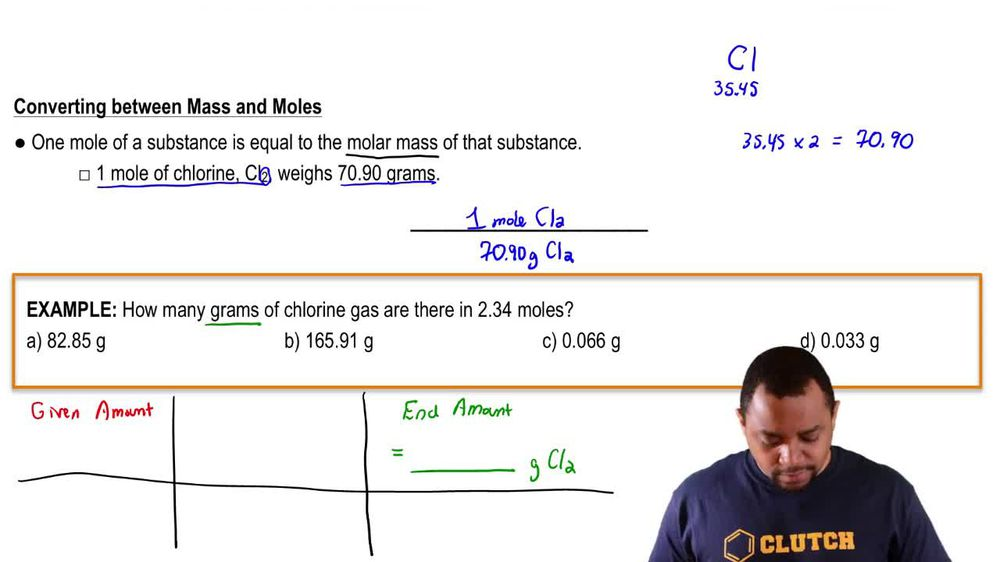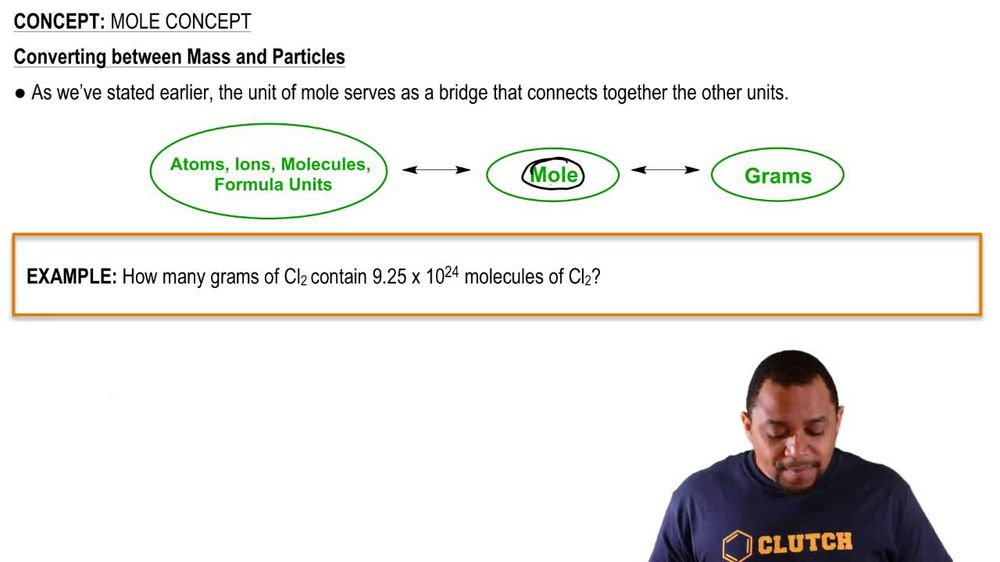Start typing, then use the up and down arrows to select an option from the list.
1. 2. Atoms & Elements2. Mole Concept
Problem

# What is the mass in grams of each of the following samples? (b) 0.0015 mol of lead

Relevant Solution1m
Play a video:
Hey everyone were asked how much does 32.6 mol of Krypton weigh in g. To do this conversion. We're first going to need to obtain the molar mass of krypton. When we look at our periodic table, we find that we have 83.8 g of krypton per one mole of Krypton. So taking our 32.6 mole of Krypton, we're going to use our dimensional analysis and our molar mass tells us that we have 83.8 g of Krypton per one mole of Krypton. So when we calculate this out and cancel out our units, we end up with a value of 2. times 10 to the 3rd g of Krypton, which is going to be our final answer. So I hope this made sense and let us know if you have any questions.